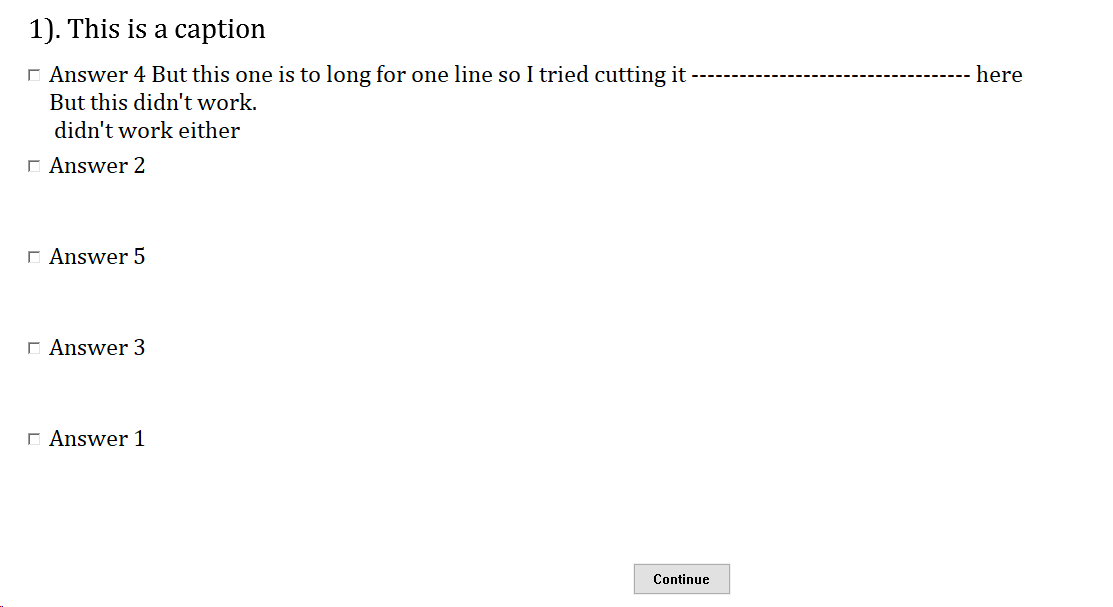Millisecond Forums

## checklist line break

https://forums.millisecond.com/Topic2252.aspx

 By buttons - 11/20/2008 Hi againJust wondering if there is anyway to insert a line break in the answers to checklist survey questions?i've tried the special charaters, but they dont seem to work.thanks By Dave - 11/20/2008 No need for special characters in this case, I think. Try it like this:/ caption="There are linebreaks in these response options:"/ required = true/ options=("This is thefirst response", "This is the second response", "This is the third response")Best,~Dave By buttons - 11/24/2008 HiUnfortunatly I've tried that and it doesn't work. Just comes up with a □ in the middle of the line.Any other ideas? By Dave - 11/24/2008 Very odd. I've double-checked and on my machine the linebreaks work as desired (see attached screenshot). Which version of Inquisit are you running (I'm at 3.0.3.1 from November 11)? You might also want to attach your non-working script here. I'd be happy to take a look and see if I can figure out what's going on.Regards,~Dave By seandr - 11/25/2008 I think Dave's hunch is correct as support for multiline button captions was added within the last couple of version updates. Try this downloading the latest update (3.0.3.1), and it should work.-Sean By Danielx - 3/16/2019 Hi Forum,I think this option does not work with/ order = randomAm I doing something wrong?Thanks in advance and have a nice dayCode:/ questions = [1 = test_cb]/ caption = "This is a caption"/ options = ("Answer 1","Answer 2","Answer 3","Answer 4 But this one is to long for one line so I tried cutting it ----------------------------------- hereBut this didn't work. ~n didn't work either""Answer 5")/ optionvalues = ("1", "2", "3", "4", "5")/ order = random/ position = (15%, 25%)/ fontstyle = ("Cambria", 3.19%, false, false, false, false, 5, 1)/ responsefontstyle = ("Cambria", 2.69%, false, false, false, false, 5, 1)/ required = falseDisplayed:By Dave - 3/17/2019 +xDanielx - Sunday, March 17, 2019Hi Forum,I think this option does not work with/ order = randomAm I doing something wrong?Thanks in advance and have a nice dayCode:/ questions = [1 = test_cb]/ caption = "This is a caption"/ options = ("Answer 1","Answer 2","Answer 3","Answer 4 But this one is to long for one line so I tried cutting it ----------------------------------- hereBut this didn't work. ~n didn't work either""Answer 5")/ optionvalues = ("1", "2", "3", "4", "5")/ order = random/ position = (15%, 25%)/ fontstyle = ("Cambria", 3.19%, false, false, false, false, 5, 1)/ responsefontstyle = ("Cambria", 2.69%, false, false, false, false, 5, 1)/ required = falseDisplayed:I don't think you're doing anything wrong,  this looks like a bug. The space required for each option is allocated according to the order in which the options are given, and when the order is randomized, the wrong amount of space is assigned to the wrong option. E.g. in your example, the space reserved for Answer 4, the long one with line breaks, is assigned to Answer 3, which is in Answer 4's expected place (wihtout randomization). The only immediate workaround I can think of would be to force an equal amount of space for each option, i.e./ questions = [1 = test_cb]/ caption = "This is a caption"/ options = ("Answer 1~n~n~t~t~t~t~t~t~t~t~t~t","Answer 2~n~n~t~t~t~t~t~t~t~t~t~t","Answer 3~n~n~t~t~t~t~t~t~t~t~t~t","Answer 4 But this one is to long for one line so I tried cutting it ----------------------------------- hereBut this didn't work. ~n didn't work either","Answer 5~n~n~t~t~t~t~t~t~t~t~t~t")/ optionvalues = ("1", "2", "3", "4", "5")/ order = random/ position = (15%, 25%)/ fontstyle = ("Cambria", 3.19%, false, false, false, false, 5, 1)/ responsefontstyle = ("Cambria", 2.69%, false, false, false, false, 5, 1)/ required = falseBy Danielx - 3/17/2019 +xDave - Monday, March 18, 2019+xDanielx - Sunday, March 17, 2019Hi Forum,I think this option does not work with/ order = randomAm I doing something wrong?Thanks in advance and have a nice dayCode:/ questions = [1 = test_cb]/ caption = "This is a caption"/ options = ("Answer 1","Answer 2","Answer 3","Answer 4 But this one is to long for one line so I tried cutting it ----------------------------------- hereBut this didn't work. ~n didn't work either""Answer 5")/ optionvalues = ("1", "2", "3", "4", "5")/ order = random/ position = (15%, 25%)/ fontstyle = ("Cambria", 3.19%, false, false, false, false, 5, 1)/ responsefontstyle = ("Cambria", 2.69%, false, false, false, false, 5, 1)/ required = falseDisplayed:I don't think you're doing anything wrong,  this looks like a bug. The space required for each option is allocated according to the order in which the options are given, and when the order is randomized, the wrong amount of space is assigned to the wrong option. E.g. in your example, the space reserved for Answer 4, the long one with line breaks, is assigned to Answer 3, which is in Answer 4's expected place (wihtout randomization). The only immediate workaround I can think of would be to force an equal amount of space for each option, i.e./ questions = [1 = test_cb]/ caption = "This is a caption"/ options = ("Answer 1~n~n~t~t~t~t~t~t~t~t~t~t","Answer 2~n~n~t~t~t~t~t~t~t~t~t~t","Answer 3~n~n~t~t~t~t~t~t~t~t~t~t","Answer 4 But this one is to long for one line so I tried cutting it ----------------------------------- hereBut this didn't work. ~n didn't work either","Answer 5~n~n~t~t~t~t~t~t~t~t~t~t")/ optionvalues = ("1", "2", "3", "4", "5")/ order = random/ position = (15%, 25%)/ fontstyle = ("Cambria", 3.19%, false, false, false, false, 5, 1)/ responsefontstyle = ("Cambria", 2.69%, false, false, false, false, 5, 1)/ required = falseOkay.Thank you for the workaround :)